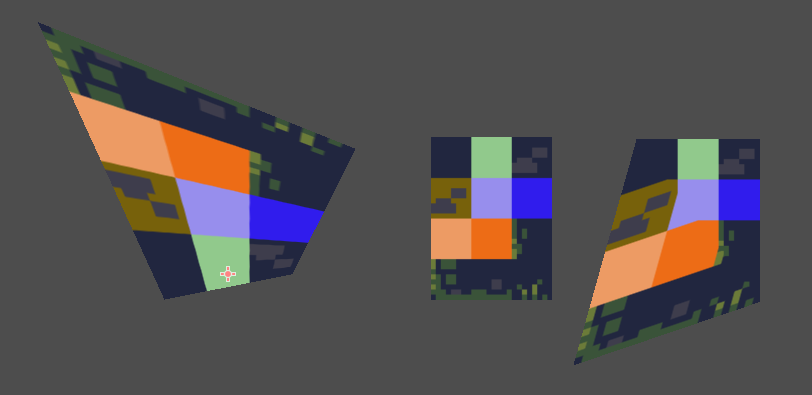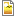# Meshing with a Mesh

In trying to answer a Godot G&A about distorting a Sprite/Mesh I came up with a (partly) solution

Using a MeshInstance2D with subdivisions it worked out as expected. But a Sprite has only 2 `triagles` forming a `quad` so moving a point influences maybe only 1 triangle with distortion on its bounderies.``````shader_type canvas_item;

// naming in UV system _x_y
uniform vec2 offset_0_0 = vec2(0.0, 0.0);
uniform vec2 offset_0_1 = vec2(0.0, 0.0);
uniform vec2 offset_1_0 = vec2(0.0, 0.0);
uniform vec2 offset_1_1 = vec2(0.0, 0.0);

vec2 sample_point(vec2 point, vec2 scale) {
// Corners for UV.y == 0
vec2 p_0_0 = vec2(0,0) - offset_0_0;
vec2 p_1_0 = vec2(1,0) - offset_1_0;
// Get point along line UV.y == 0
vec2 d_x_0 = p_0_0 + (p_1_0 - p_0_0) * point.x;

// Corners for UV.y == 1.0
vec2 p_0_1 = scale * ( vec2(0, 1) - offset_0_1);
vec2 p_1_1 = vec2(1, 1) - offset_1_1;
// Get point along line UV.y == 1
vec2 d_x_1 = p_0_1 + (p_1_1 - p_0_1) * point.x;
// Get point for given UV.y
return d_x_0 + (d_x_1 - d_x_0) * point.y;
}

void fragment() {
//COLOR = vec4(UV.x, UV.y, .0, 1.0);
//COLOR = texture(TEXTURE, UV);
//COLOR = texture(TEXTURE, sample_point(UV, vec2(.01, .01)));
}

void vertex() {
VERTEX += sample_point(UV, vec2(1,1));
}
``````On the left MeshInstance2D with in the middle original sprite and distorted sprite.

AttachmentSizegodot-q-a-92415.png38.83 KBgodot-q-a-92415-triangle.png41.67 KB